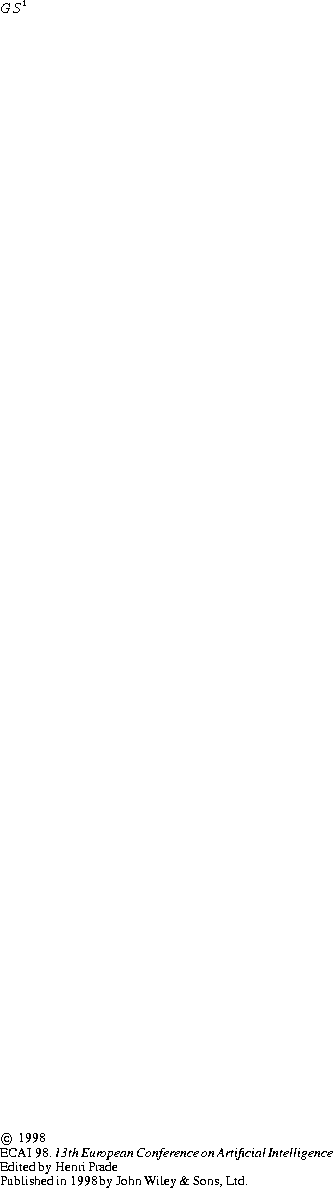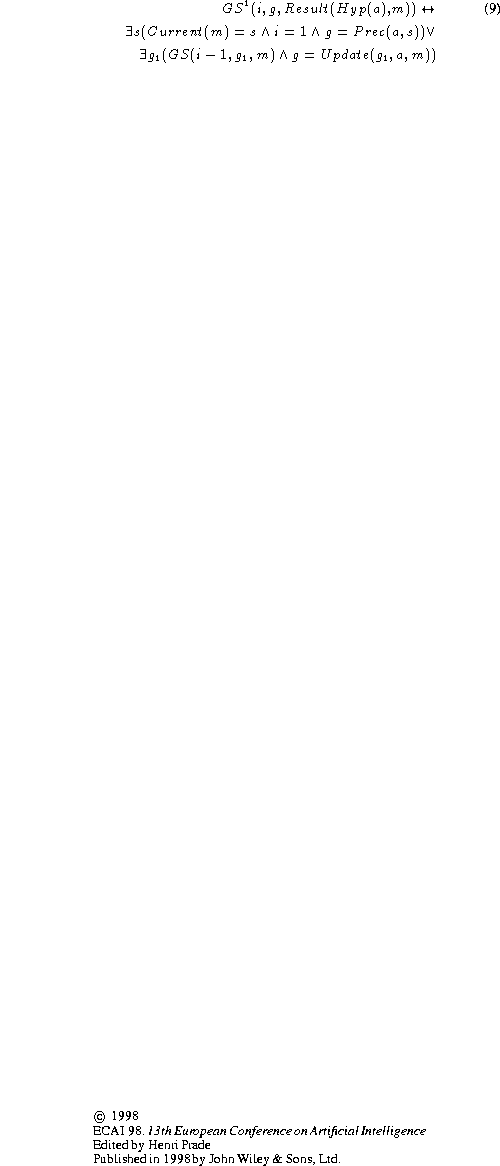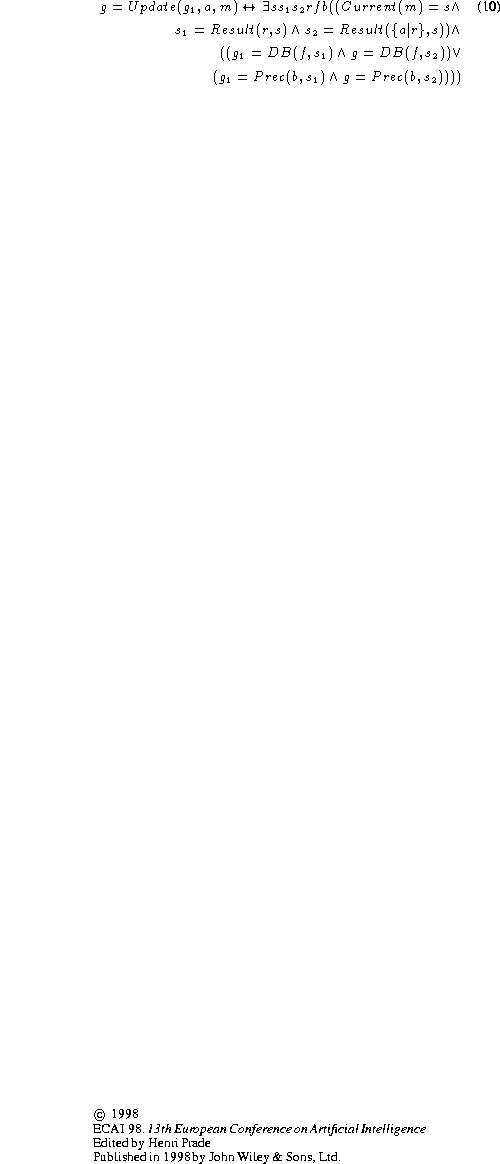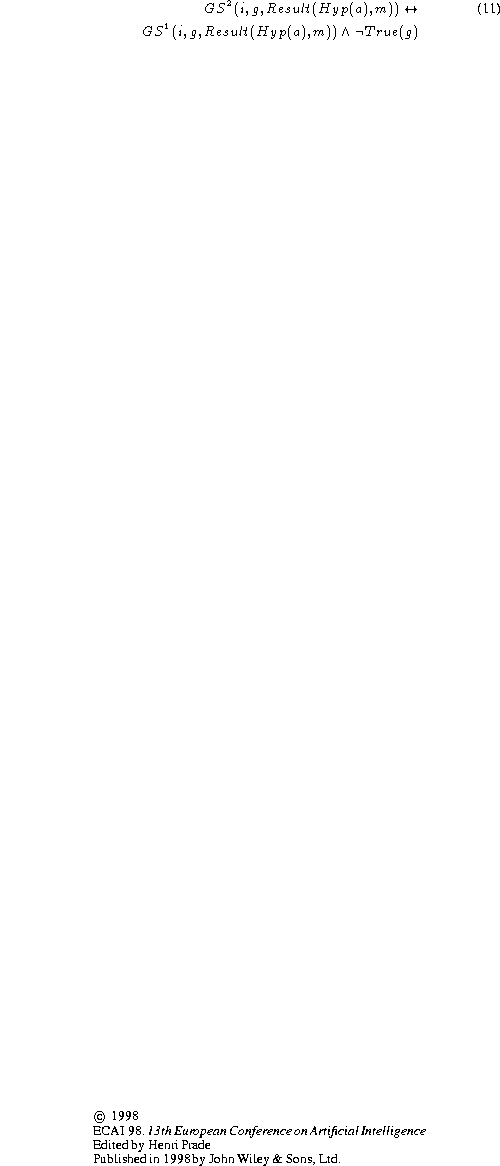Next: Appropriateness Up: THE REASONING STRATEGY Previous: Mental Situations

## Mental Actions

Axiomstodescribe the effects of the mental action Hyp(a) -which summarizes the three-step cycle of the algorithm- on the fluents that characterize a mental situation, GS(i,f,m) and Current(m). The expression Result(Hyp(a),m) denotes the mental situation resulting from performing Hyp(a) in mental situation m. The predicatesanddescribe the intermediate goal stacks resulting from steps 1 and 2, respectively.

The effect of step 1 is formalized as follows. A subgoal describing the fact that the precondition of action a should hold at the current situation associated with m is added to the top (location 1) of the goal stack. The location numbers of the subgoals that were in the goal stack associated with m are incremented by 1. Instead of adding action a to the goal stack, we update the situation arguments of the subgoalsthat were in the goal stack associated with m. The idea is to take into account the fact that adding action a to the top of the goal stack associated with m is equivalent to hypothesizing the execution of a in the current situation associated with m.The sequences of actions associated with the situation arguments of the subgoals in the goal stack associated with a mental situation describe the plan (or a part of the plan) that is being constructed by STRIPS in the process of searching for a solution. Hyp(a) denotes the mental act of hypothesizing that inserting action a after the sequence of actions that have been applied to the database describing the current situation may improve the plan constructed so far. This can be formalized by modifying the situation arguments of the subgoals according to the following update function. The function Update maps a subgoal of the forminto a subgoal of the form, whereis obtained fromas follows. If s is the current situation associated with m andis the situation resulting of executing the sequence of actions r in s (i.e.,), thenis the situation resulting of executing the sequence of actions r preceded by action a in s (i.e.,). Update maps a subgoal of the forminto a subgoal of the form, whereis obtained fromas explained above.The effect of step 2 is formalized as follows. True subgoals are popped fromyielding.Ifis not empty the situation argument of the top subgoal ofis taken as the new current situation. Ifis empty, the situation argument of the last subgoal popped fromis taken as the current situation. Axiomsandcapture the intuition that a subgoal is popped from the goal stack iff it has been satisfied by the current database at some point during the process of popping off true subgoals. Therefore, the sequence of actions associated with the situation argument of the subgoal must have been applied to DB at that point.Finally, the effect of step 3 is formalized as follows. Ifis not empty, then the goal stack GS associated with Result(Hyp(a),m) is. Otherwise, a subgoal of the formis added to GS for each formula f in the stack describing the problem's goal situation that is not true in the current situation.Next: Appropriateness Up: THE REASONING STRATEGY Previous: Mental Situations

Josefina Sierra
Tue Jul 21 09:26:01 PDT 1998